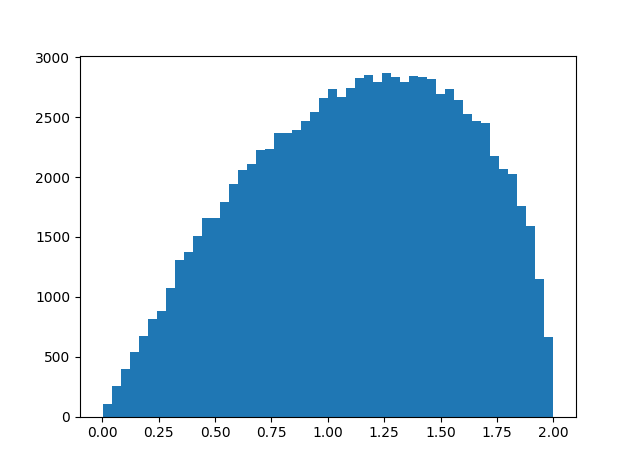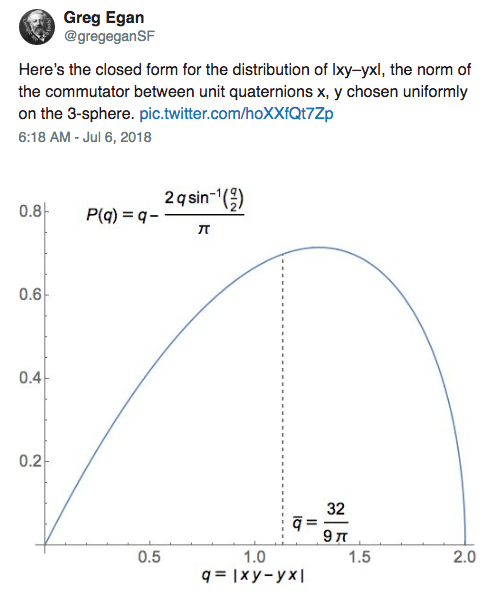Over a million developers have joined DZone.{{announcement.body}}
{{announcement.title}}

DZone's Guide to

# How Far Is xy From yx on Average for Quaternions?

In this post, a math whiz and developer demonstrates how to work with Python to create some complex data models. Read on to get started!

· Big Data Zone ·
Free Resource

Comment (0)

Save
{{ articles.views | formatCount}} Views

The open source HPCC Systems platform is a proven, easy to use solution for managing data at scale. Visit our Easy Guide to learn more about this completely free platform, test drive some code in the online Playground, and get started today.

Given two quaternions x and y, the product xy might equal the product yx, but, in general, the two results are different.

How different are xy and yx on average? That is, if you selected quaternions x and y at random, how big would you expect the difference xy - yx to be? Since this difference would increase proportionately if you increased the length of x or y, we can just consider quaternions of norm 1. In other words, we're looking at the size of xy - yx relative to the size of xy.

Here's simulation code to explore our question.

``````    import numpy as np

def random_unit_quaternion():
x = np.random.normal(size=4)
return x / np.linalg.norm(x)

def mult(x, y):
return np.array([
x*y - x*y - x*y - x*y,
x*y + x*y + x*y - x*y,
x*y - x*y + x*y + x*y,
x*y + x*y - x*y + x*y
])

N = 10000
s = 0
for _ in range(N):
x = random_unit_quaternion()
y = random_unit_quaternion()
s += np.linalg.norm(mult(x, y) - mult(y, x))

print(s/N)``````

In this code, x and y have unit length, and so xy and yx also have unit length. Geometrically, x, y, xy, and yx are points on the unit sphere in four dimensions.

When I ran the simulation above, I got a result of 1.13, meaning that on average xy and yx are further from each other than they are from the origin.

To see more than the average, here's a histogram of || xy - yx|| with `N` above increased to 100,000.I imagine you could work out the distribution exactly, though it was quicker and easier to write a simulation. We know the distribution lives on the interval [0, 2] because xy and yx are points on the unit sphere. Looks like the distribution is skewed toward its maximum value, and so xy and yz are more likely to be nearly antipodal than nearly equal.

Update: Greg Egan worked out the exact mean and distribution.Managing data at scale doesn’t have to be hard. Find out how the completely free, open source HPCC Systems platform makes it easier to update, easier to program, easier to integrate data, and easier to manage clusters. Download and get started today.

Topics:
probability and statistics ,big data ,quaternions ,python

Comment (0)

Save
{{ articles.views | formatCount}} Views

Published at DZone with permission of

Opinions expressed by DZone contributors are their own.

# {{ parent.title || parent.header.title}}

{{ parent.tldr }}

{{ parent.urlSource.name }}